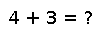# airyx

airyx(k,x)

scaled kth derivative of the Airy function, return $\operatorname{Ai}(x) e^{\frac{2}{3} x \sqrt{x}}$ for k == 0 || k == 1, and $\operatorname{Ai}(x) e^{- \left| \operatorname{Re} \left( \frac{2}{3} x \sqrt{x} \right) \right|}$ for k == 2 || k == 3.

## Examples

Checking you are not a robot: